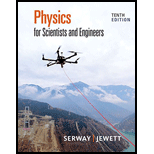Chapter 16, Problem 1PPhysics for Scientists and Enginee...

10th Edition
Raymond A. Serway + 1 other
ISBN: 9781337553278

Solutions

Chapter
SectionPhysics for Scientists and Enginee...

10th Edition
Raymond A. Serway + 1 other
ISBN: 9781337553278
Textbook Problem

A seismographic station receives S and P waves from an earthquake, separated in time by 17.3 s. Assume the waves have traveled over the same path at speeds of 4.30 km/s and 7.80 km/s. Find the distance from the seismograph to the focus of the quake.

To determine

The distance from the seismograph to the focus of the quake.

The distance from the seismograph to the focus of the quake is 184km.

Explanation

The time separation between the S and P waves received by seismographic station is 17.3s, the speed of the S wave is 4.50km/s and the speed of the P wave is 7.80km/s.

Formula to calculate the distance travelled by P wave is,

d=vpt        (I)

Here, d is the distance travelled by P wave, vp is the speed of P wave and t is the time taken by P wave.

Formula to calculate the distance travelled by the S wave is,

d=vs(t+17.3s)        (II)

Here, d is the distance travelled by S wave and vs is the speed of S wave.

Equate equation (I) and (II).

vpt=vs(t+17.3s)(vpvs)t=(17.3s)vst=(17.3s)vs(vpvs)

Substitute (17.3s)vs(vpvs) for t in equation (I).

d=(17.3s)vsvp(vpvs)

Substitute 7.80km/s for vp and 4.50km/s for vs to find d.

d=(17.3s)(4.50km/s)(7.80km/s)(7.80km/s4.50km/s)=184km

Conclusion:

Therefore, the distance from the seismograph to the focus of the quake is 184km.

The Solution to Your Study Problems

Bartleby provides explanations to thousands of textbook problems written by our experts, many with advanced degrees!

Get Started

Give the names of the following ions. a Cr3+ b Pb4+ c Ti2 d Cu2+

General Chemistry - Standalone book (MindTap Course List)

How is a compact object different from a star?

Foundations of Astronomy (MindTap Course List)

What are the starting products for photosynthesis? The end products?

Oceanography: An Invitation To Marine Science, Loose-leaf Versin

Find all the answers to your study problems with bartleby.
Textbook solutions plus Q&A. Get As ASAP arrow_forward